# 森林野火故事2.0：一眼看穿！使用 Panel和hvPlot可视化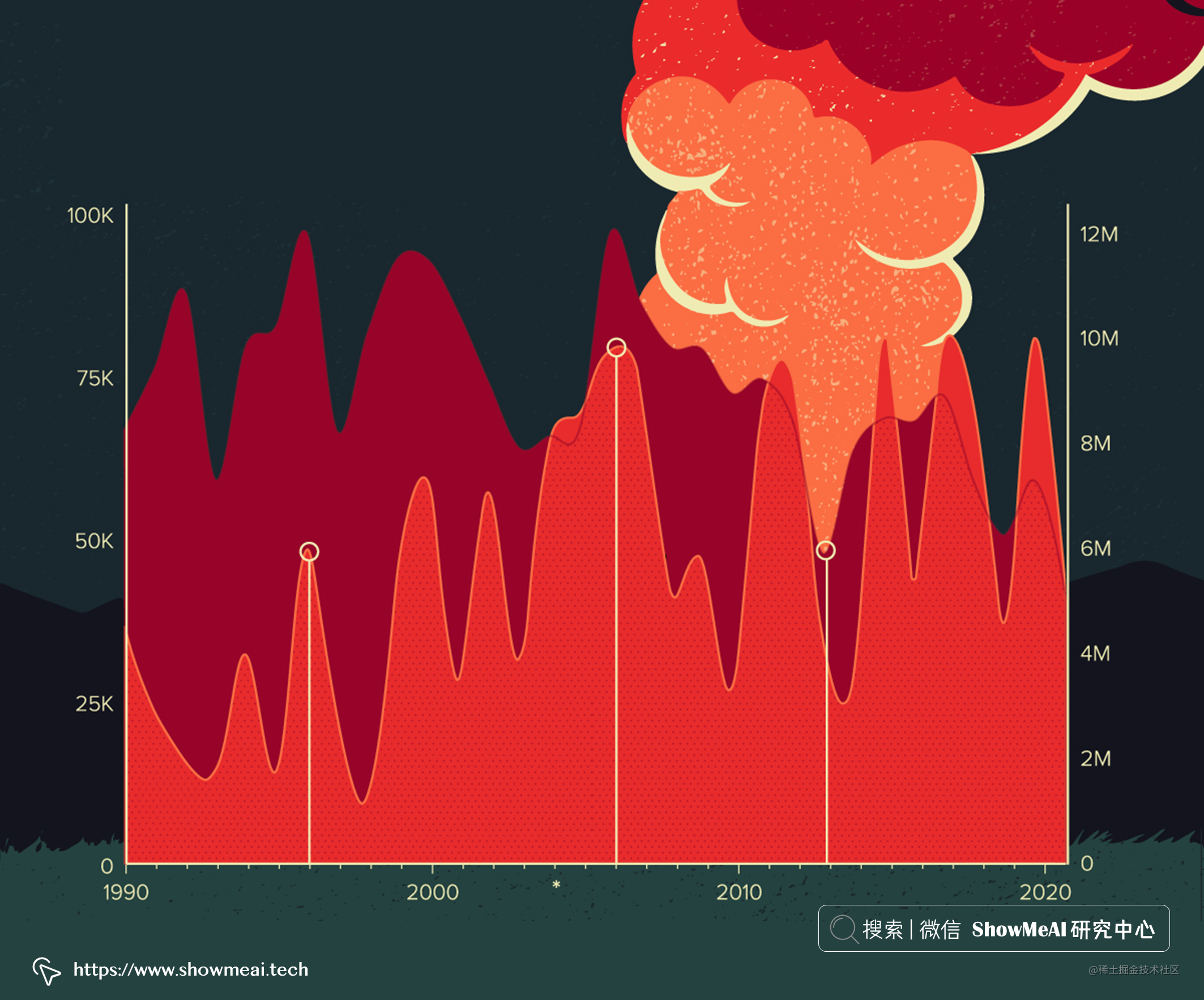📘Panel 是 Python 中一个非常棒的可以用作制作数据仪表板的工具库，基于它可以轻松构建数据可视化看板。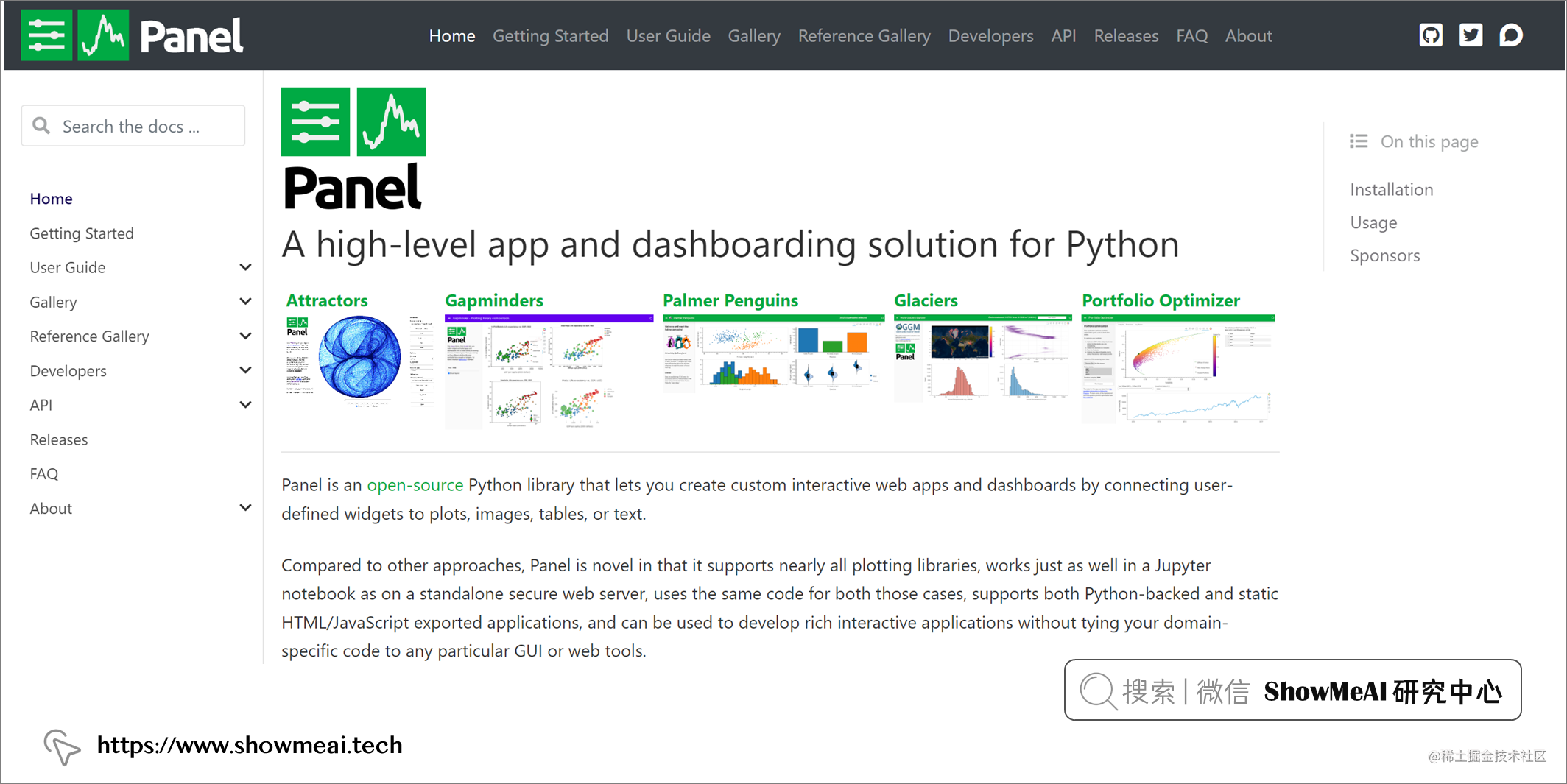# 💡导入工具库

``````# 数据库
import sqlite3

# 数据处理
import numpy as np
import pandas as pd

# 可视化与仪表盘
import holoviews as hv
import colorcet as cc
import panel as pn

from holoviews.element.tiles import EsriImagery
from datashader.utils import lnglat_to_meters
import hvplot.pandas
hv.extension('bokeh')

# 💡数据准备

🏆 实战数据集下载（百度网盘）：公众号『ShowMeAI研究中心』回复『实战』，或者点击 这里 获取本文 基于Panel和hvPlot的可视化交互看板实战案例美国野火 FPA_FOD_20170508.sqlite 数据集

ShowMeAI官方GitHubgithub.com/ShowMeAI-Hu…

``````# 连接数据库
conn = sqlite3.connect('../data/FPA_FOD_20170508.sqlite')

# 选取信息：经纬度、原因描述、火势大小与等级、日期、所在州、年份 等
df = pd.read_sql_query("SELECT LATITUDE, LONGITUDE, STAT_CAUSE_DESCR, FIRE_SIZE, FIRE_SIZE_CLASS, DISCOVERY_DATE, CONT_DATE, STATE, FIRE_YEAR FROM fires", conn)

# 数据中有一些不在美国的记录，删除它们
df = df.loc[(df.loc[:,'STATE']!='AK') & (df.loc[:,'STATE']!='HI') & (df.loc[:,'STATE']!='PR')]

# 计算野火持续时间
df['BURN_TIME'] = df['CONT_DATE'] - df['DISCOVERY_DATE']

# 查看数据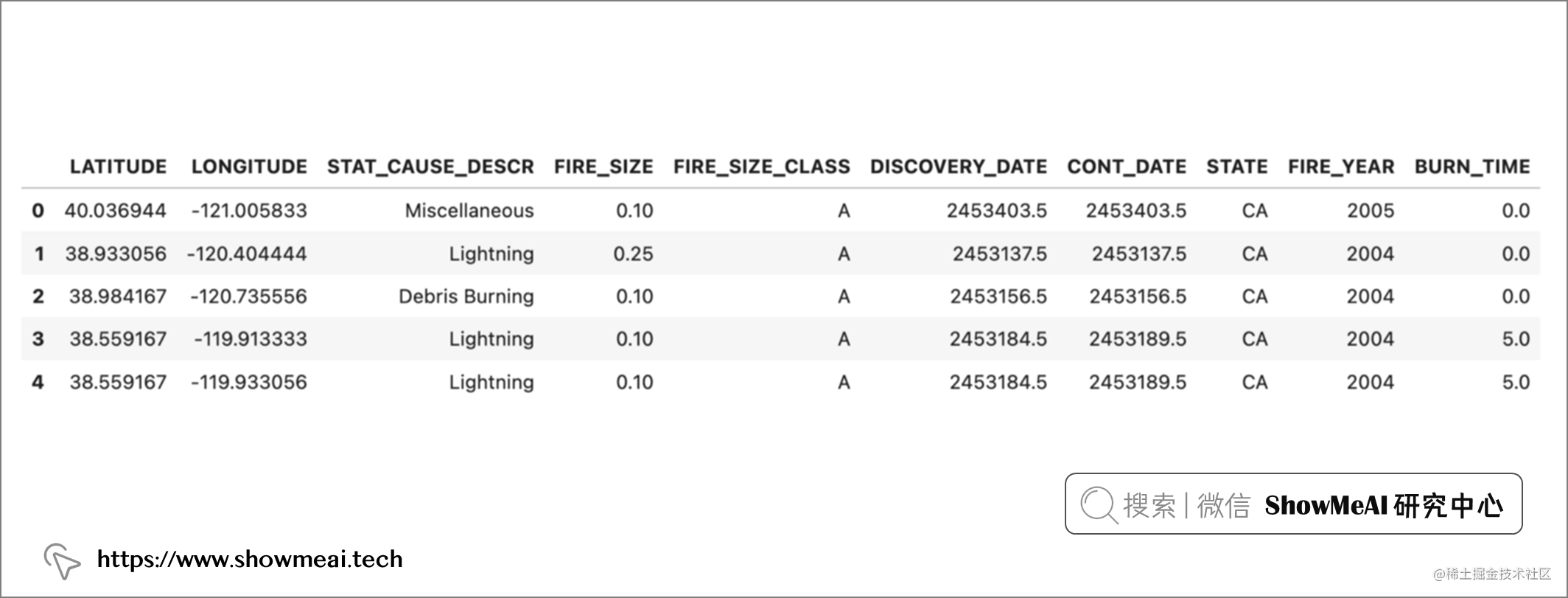# 💡野火地图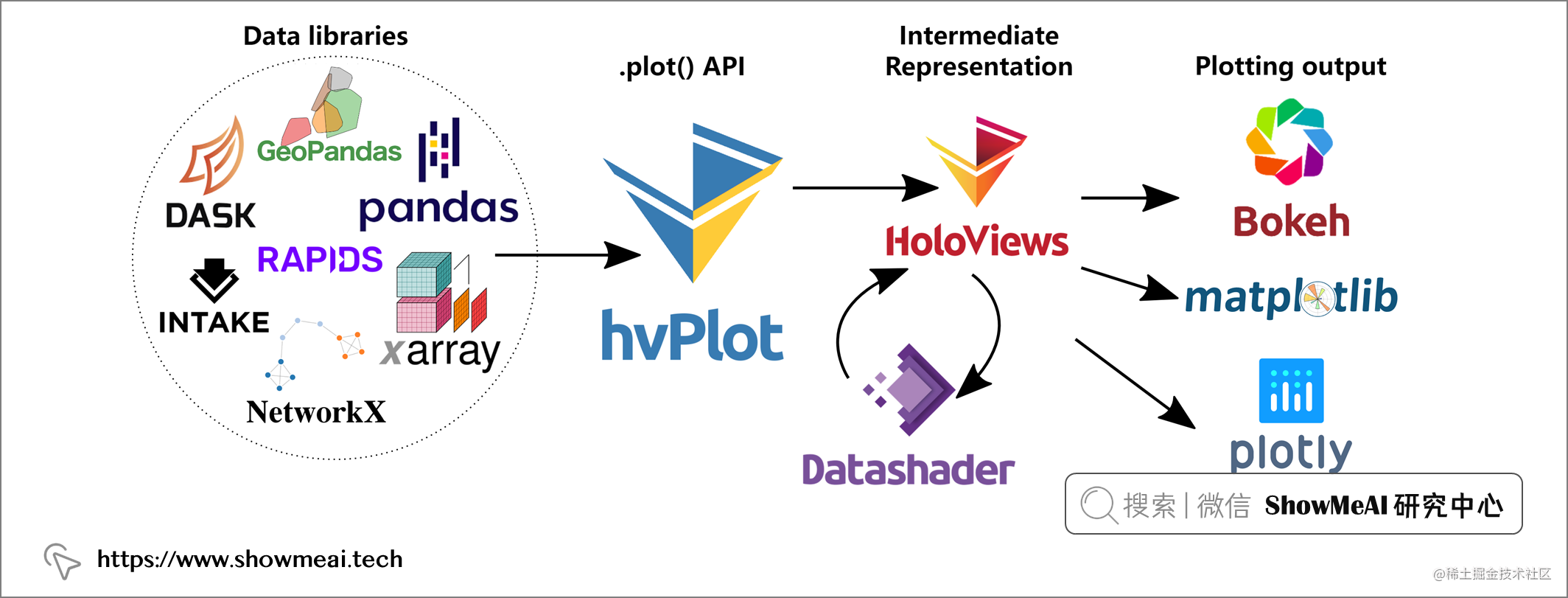``````map_tiles = EsriImagery().opts(alpha=0.5, width=700, height=480, bgcolor='black')
plot = df.hvplot(
'LONGITUDE',
'LATITUDE',
geo=True,
kind='points',
rasterize=True,
cmap=cc.fire,
cnorm='eq_hist',
colorbar=True).opts(colorbar_position='bottom', xlabel='', ylabel='')
map_tiles * plot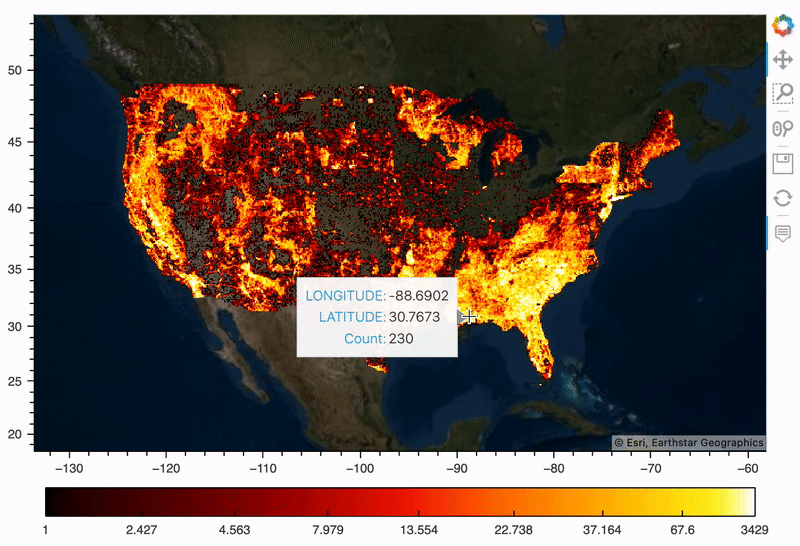# 💡带时间滑块的仪表板

Panel 的小部件让我们可以访问各种方法来操作和切分我们的数据子集可视化，例如对时间序列数据可切分：加入选择年份的滑块

• ① 定义一个小部件，例如用于选择年份或下拉列表的整数滑块。
• ② 定义一个绘图函数，将滑块中的年份值作为输入。
• ③ 布局和渲染我们的仪表板。
``````# 为时间序列构建滑动小组件
year = pn.widgets.IntSlider(name='Year Slider', width=300,
start=1992, end=2015, value=(1993),
step=1,value_throttled=(1993))

# 展示当前年份
@pn.depends(year.param.value_throttled)
def year_selected(year):
return '## Wildfires Across the US in {}'.format(year)

# 实际绘图函数
@pn.depends(year.param.value_throttled)
def plot_map(year):
year_df = df[df['FIRE_YEAR'] == year].copy()
plot = year_df.hvplot(
'LONGITUDE',
'LATITUDE',
geo=True,
kind='points',
rasterize=True,
cmap=cc.fire,
cnorm='eq_hist',
colorbar=True).opts(colorbar_position='bottom', xlabel='', ylabel='')
return map_tiles * plot

dashboard = pn.WidgetBox(pn.Column(pn.Row(year_selected, year),
pn.Row(pn.bind(plot_map, year)), align="start",
sizing_mode="stretch_width"))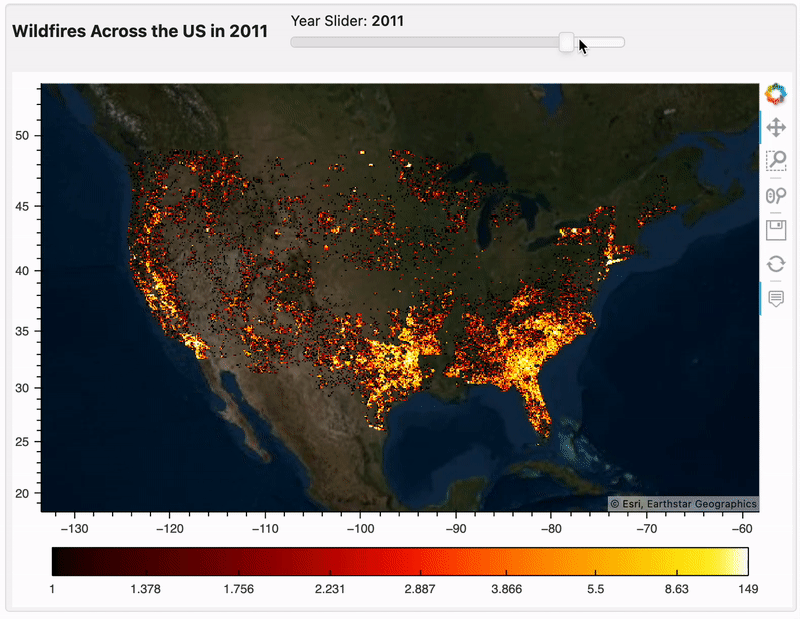# 💡更简单的构建方式

``````select_cause = pn.widgets.Select(
options = df['STAT_CAUSE_DESCR'].value_counts().index.tolist(),
name = 'Cause')

# 动态交互
dfi = df.interactive

iplot = dfi[dfi['STAT_CAUSE_DESCR']==select_cause].hvplot(
'LONGITUDE',
'LATITUDE',
geo=True,
kind='points',
rasterize=True,
cmap=cc.fire,
cnorm='eq_hist',
colorbar=True).opts(colorbar_position='bottom', xlabel='', ylabel='', title='Wildfires by Cause from 1992-2015')

map_tiles.opts(level='underlay') * iplot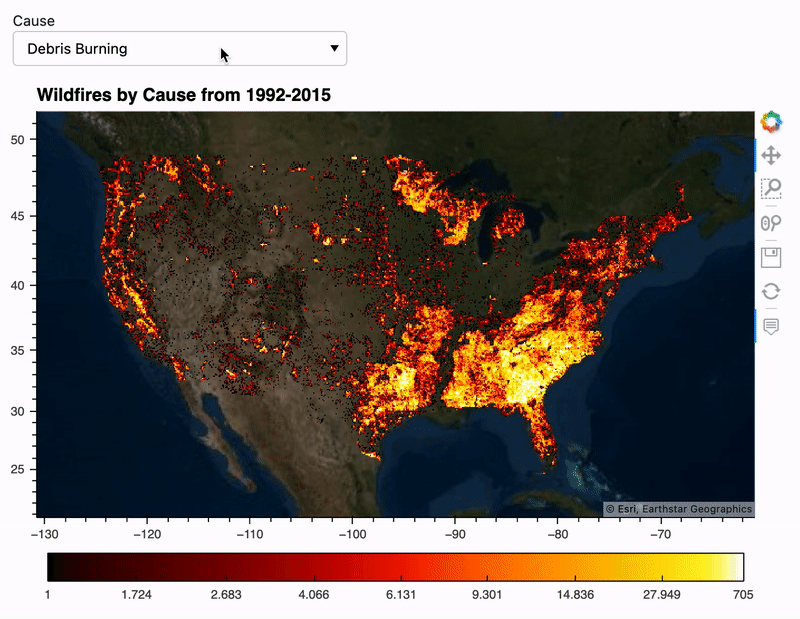# 💡组合仪表板

## 💦 火势大小

``````@pn.depends(year.param.value_throttled)
def plot_class(year):
year_df = df[df['FIRE_YEAR'] == year].copy()
count_df = pd.DataFrame(year_df.groupby('FIRE_SIZE_CLASS').size(), columns=['Count'])
count_df['Fire Class'] = count_df.index
return count_df.hvplot.bar(x='Fire Class', y='Count', c='Fire Class', cmap='fire',
legend=False).opts(xlabel="Fire Size Class", ylabel="Number of Fires",
title="Wildfires in {} Grouped by Size Classification".format(year))

plot_class(2006)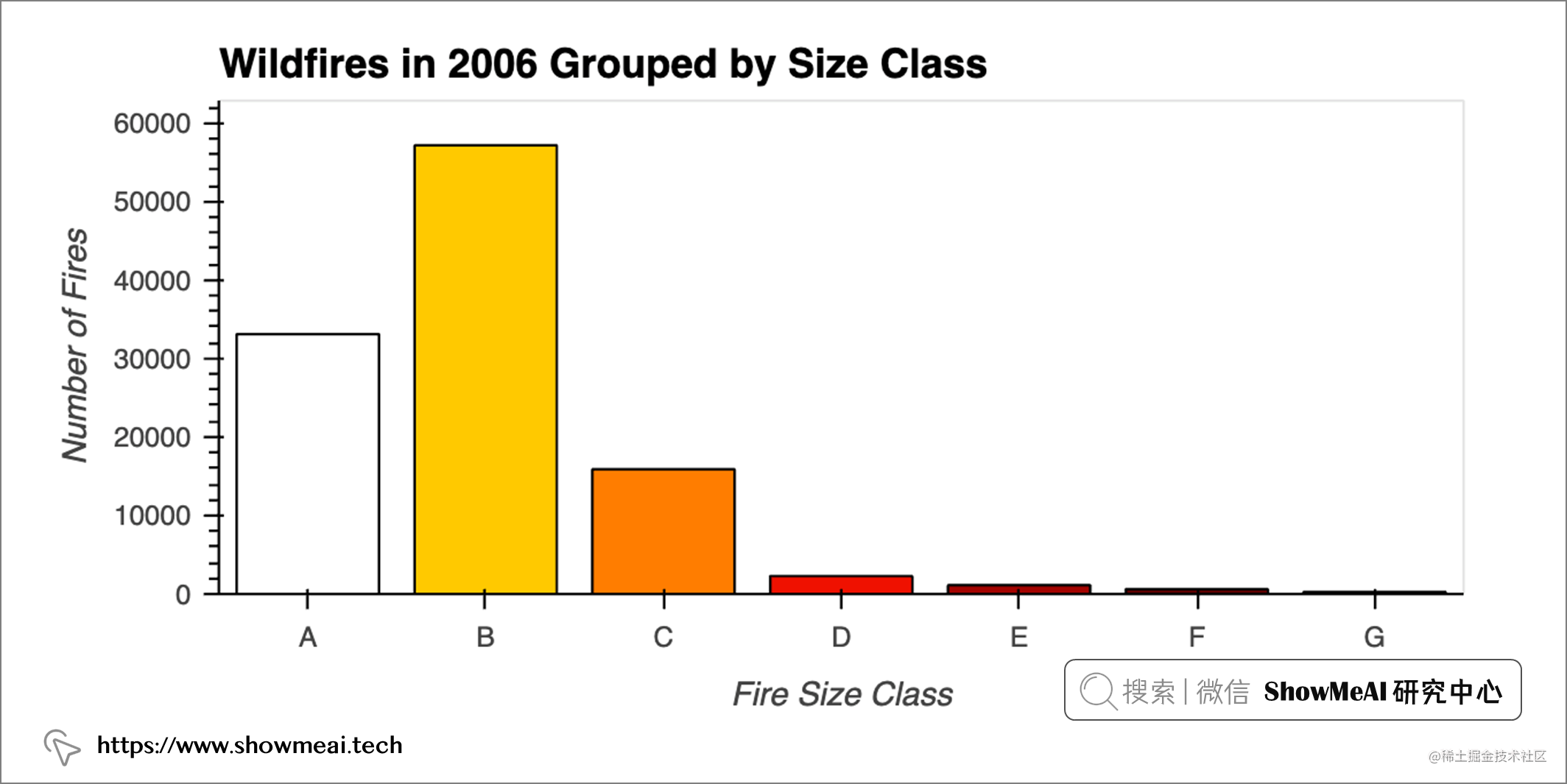## 💦 起火原因 & 持续时长

``````@pn.depends(year.param.value_throttled)
def plot_cause_burn(year):
year_df = df[df['FIRE_YEAR'] == year].copy()
caused_df = pd.DataFrame(year_df.groupby('STAT_CAUSE_DESCR')[['BURN_TIME']].mean().sort_values('BURN_TIME'))
caused_df['Fire Cause'] = caused_df.index
return caused_df.hvplot.barh(x='Fire Cause', y='BURN_TIME',
c='Fire Cause', cmap='fire_r', legend=False).opts(
ylabel="Burn Time (Days)",
title="Wildfire Burn time Grouped by Cause in {}".format(year))

plot_cause_burn(2003)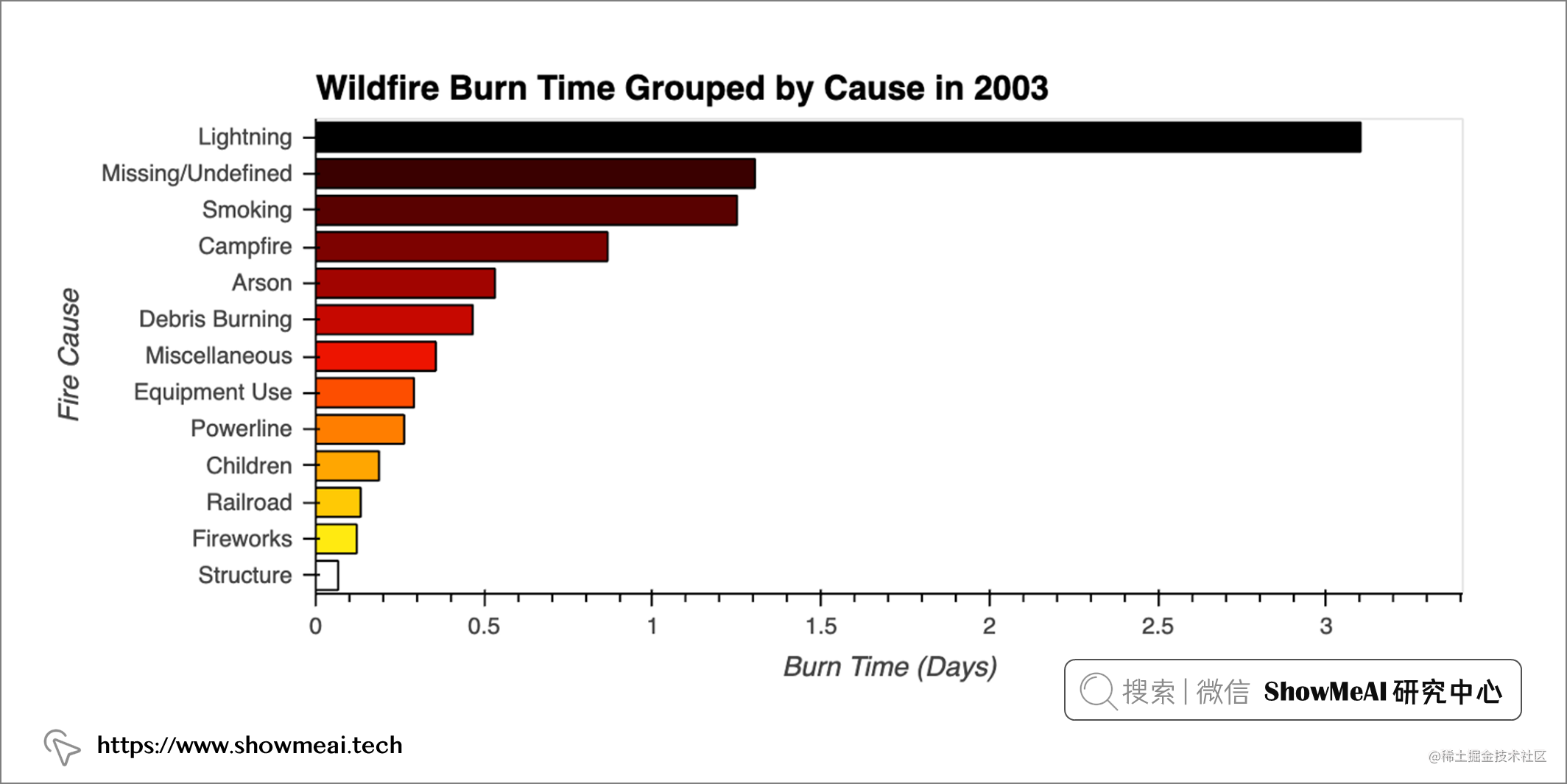## 💦 起火原因 & 火灾数量

``````@pn.depends(year.param.value_throttled)
def plot_cause_count(year):
year_df = df[df['FIRE_YEAR'] == year].copy()
caused_df = pd.DataFrame(year_df.groupby('STAT_CAUSE_DESCR').size(), columns=['Count']).sort_values('Count')
caused_df['Fire Cause'] = caused_df.index
return caused_df.hvplot.barh(x='Fire Cause', y='Count',
c='Fire Cause', cmap='fire_r',
legend=False).opts(ylabel="Number of Fires",
title="Number of Wildfires Grouped by Cause in {}".format(year))

plot_cause_count(2003)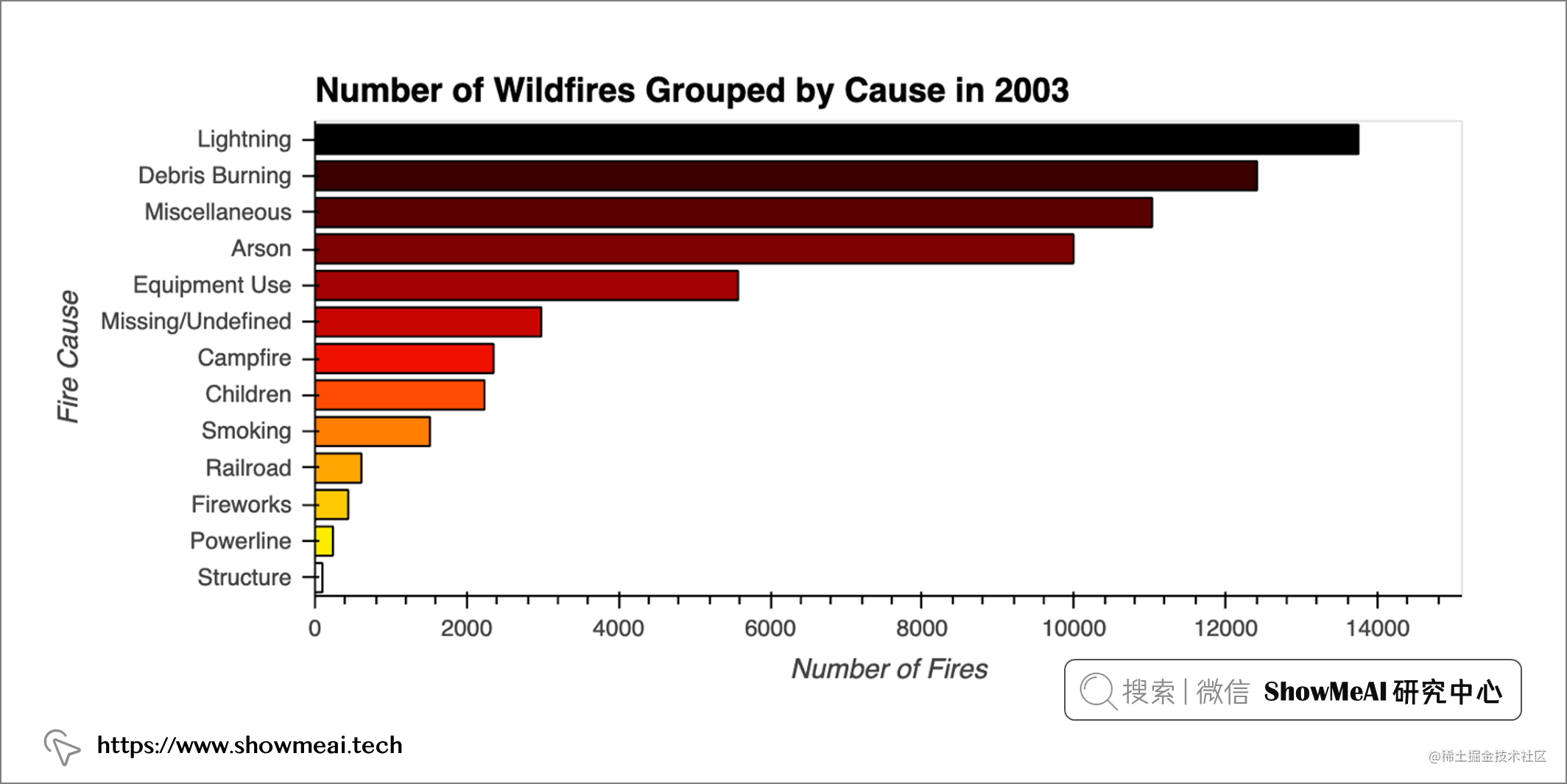• 吸烟带来第2燃烧时长的野火，而它在引发的野火总数中排名第9。
• 除了闪电之外，燃烧时间最长的火灾并不是最频繁发生的火灾。

``````pn.Row(plot_cause_count(2006) , plot_cause_burn(2006), width=1000)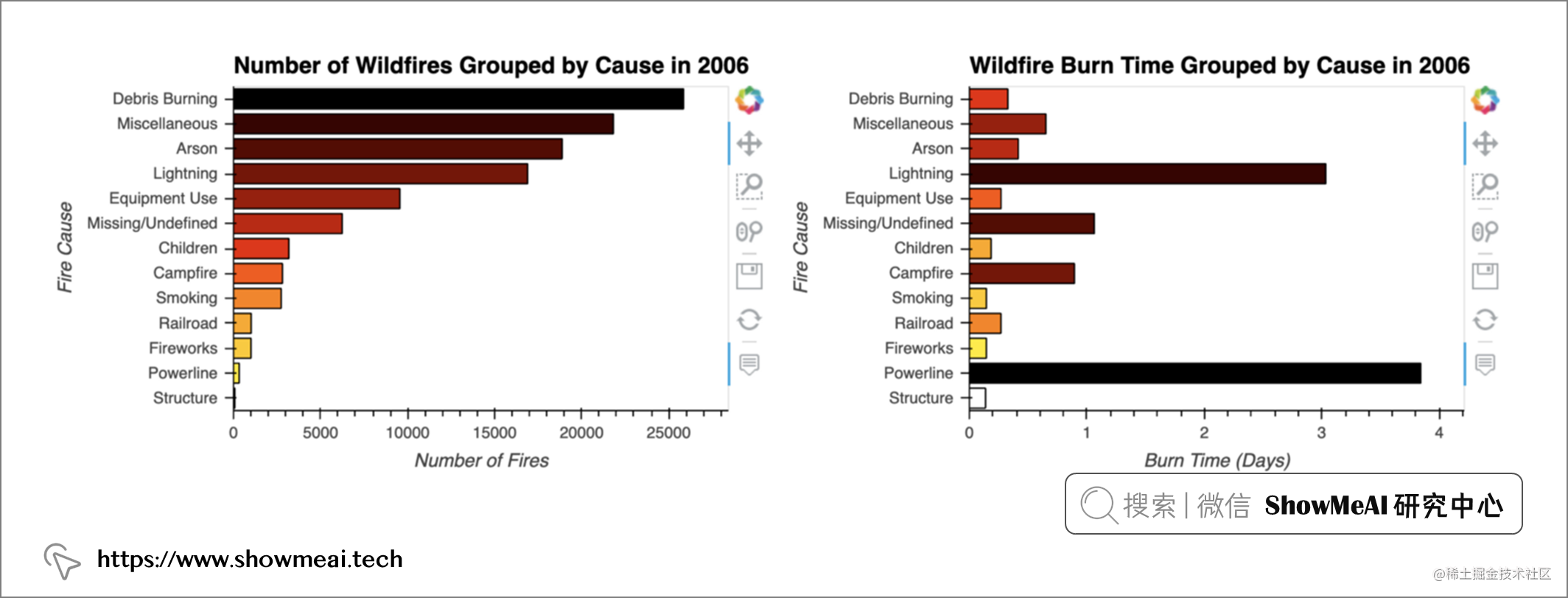## 💦 分析结果组装

``````plots_box = pn.WidgetBox(pn.Column(pn.Row(pn.bind(fire_count, year), year),
pn.Row(pn.bind(plot_map, year), pn.bind(plot_class, year)) ,
pn.Row(pn.bind(plot_cause_count, year), pn.bind(plot_cause_burn, year)), align="start",
width=800, sizing_mode="stretch_width"))

dashboard = pn.Row(plots_box, sizing_mode="stretch_width")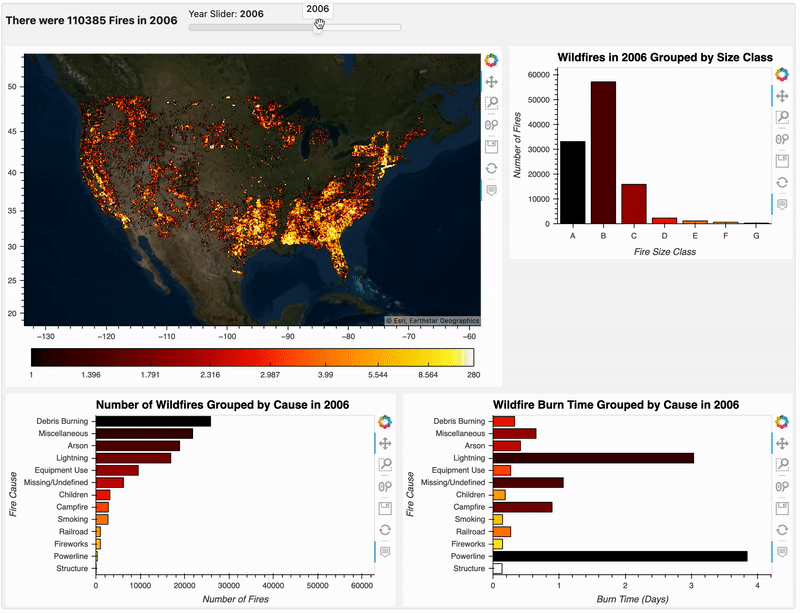# 参考资料